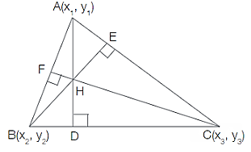# Orthocenter Formula

## Orthocenter Formula - Orthocenter of a Triangle Formulas

The three altitudes of any triangle are concurrent line segments (they intersect in a single point) and this point is known as the orthocenter of the triangle. The steps to find the coordinates of the orthocenter of a triangle are relatively simple, given that we know the coordinates of the vertices of the triangle .
Let us consider the following triangle ABC, the coordinates of whose vertices are known.First, we will find the slopes of any two sides of the triangle (say AC and BC).
${m_{AC}} = \frac{{\left( {{y_3} - {y_1}} \right)}}{{\left( {{x_3} - {x_1}} \right)}}\quad \quad {m_{BC}} = \frac{{\left( {{y_3} - {y_2}} \right)}}{{\left( {{x_3} - {x_2}} \right)}}$
Next, we can find the slopes of the corresponding altitudes. Remember that if two lines are perpendicular to each other, they satisfy the following equation.
$\begin{gathered} {m_{AC}} \times {m_{BE}} = - 1\quad \quad {m_{BC}} \times {m_{AD}} = - 1 \hfill \\ {m_{BE}} = \frac{{ - 1}}{{{m_{AC}}}}\quad \quad \,{m_{AD}} = \frac{{ - 1}}{{{m_{BC}}}} \hfill \\ \end{gathered}$
Next, we will use the slope-point form of the equation of a straight line to find the equations of the lines that are coincident with the altitudes BE and AD.
$BE:\frac{{\left( {y - {y_2}} \right)}}{{\left( {x - {x_2}} \right)}} = {m_{BE}}\quad \quad AD:\frac{{\left( {y - {y_1}} \right)}}{{\left( {x - {x_1}} \right)}} = {m_{AD}}$
Next, we can solve the equations of BE and AD simultaneously to find their solution, which gives us the coordinates of the orthocenter H.
Let’s see an example now.

Question: Find the coordinates of the orthocenter of a triangle ABC whose vertices are A(1 ,7), B(−6, 0) and C(3, 4).
Solution:
$\begin{gathered} {m_{AC}} = \frac{{\left( {{y_3} - {y_1}} \right)}}{{\left( {{x_3} - {x_1}} \right)}} = \frac{{\left( {4 - 7} \right)}}{{\left( {3 - 1} \right)}} = \frac{{ - 3}}{2} \Rightarrow {m_{BE}} = \frac{{ - 1}}{{{m_{AC}}}} = \frac{2}{3} \hfill \\ {m_{BC}} = \frac{{\left( {{y_3} - {y_2}} \right)}}{{\left( {{x_3} - {x_2}} \right)}} = \frac{{\left( {4 - 0} \right)}}{{\left( {3 - \left( { - 6} \right)} \right)}} = \frac{4}{9} \Rightarrow {m_{AD}} = \frac{{ - 1}}{{{m_{BC}}}} = \frac{{ - 9}}{4} \hfill \\ BE:\frac{{\left( {y - {y_2}} \right)}}{{\left( {x - {x_2}} \right)}} = {m_{BE}} \Rightarrow \frac{{\left( {y - 0} \right)}}{{\left( {x - \left( { - 6} \right)} \right)}} = \frac{2}{3} \Rightarrow 2x - 3y + 12 = 0 \hfill \\ AD:\frac{{\left( {y - {y_1}} \right)}}{{\left( {x - {x_1}} \right)}} = {m_{AD}} \Rightarrow \frac{{\left( {y - 7} \right)}}{{\left( {x - 1} \right)}} = \frac{{ - 9}}{4} \Rightarrow 9x + 4y - 37 = 0 \hfill \\ \end{gathered}$
Solving the equations for BE and AD , we get the coordinates of the orthocenter H as follows.
$H\left( {\frac{9}{5},\frac{{26}}{5}} \right)$
Why don’t you try to solve a problem to see if you are getting the hang of the methodology?

Question:
Find the coordinates of the orthocenter of a triangle ABC whose vertices are A (−1, −4), B (2, −3) and C (5, 2).
Options:
(a) (10, 9)
(b) (10, −9)
(c) (9, 10)
(d) (9, −10)
$\begin{gathered} {m_{AC}} = \frac{{\left( {{y_3} - {y_1}} \right)}}{{\left( {{x_3} - {x_1}} \right)}} = \frac{{\left( {2 - \left( { - 4} \right)} \right)}}{{\left( {5 - \left( { - 1} \right)} \right)}} = 1 \Rightarrow {m_{BE}} = \frac{{ - 1}}{{{m_{AC}}}} = - 1 \hfill \\ {m_{BC}} = \frac{{\left( {{y_3} - {y_2}} \right)}}{{\left( {{x_3} - {x_2}} \right)}} = \frac{{\left( {2 - \left( { - 3} \right)} \right)}}{{\left( {5 - 2} \right)}} = \frac{5}{3} \Rightarrow {m_{AD}} = \frac{{ - 1}}{{{m_{BC}}}} = \frac{{ - 3}}{5} \hfill \\ BE:\frac{{\left( {y - {y_2}} \right)}}{{\left( {x - {x_2}} \right)}} = {m_{BE}} \Rightarrow \frac{{\left( {y - \left( { - 3} \right)} \right)}}{{\left( {x - 2} \right)}} = - 1 \Rightarrow x + y + 1 = 0 \hfill \\ AD:\frac{{\left( {y - {y_1}} \right)}}{{\left( {x - {x_1}} \right)}} = {m_{AD}} \Rightarrow \frac{{\left( {y - \left( { - 4} \right)} \right)}}{{\left( {x - \left( { - 1} \right)} \right)}} = \frac{{ - 3}}{5} \Rightarrow 3x + 5y + 23 = 0 \hfill \\ \end{gathered}$
$H\left( {9, - 10} \right)$